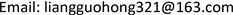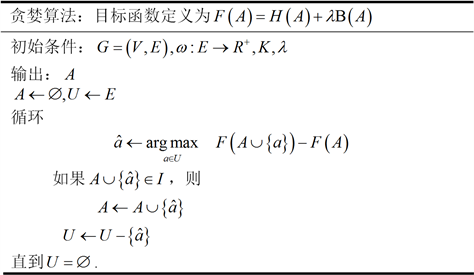﻿ 拟阵约束下最大化子模函数的模型及其算法的一种熵聚类方法 An Entropy Clustering Method for the Model and Its Algorithm of the Maximizing a Submodular Function Subject to a Matroid Constraint

Computer Science and Application
Vol.07 No.10(2017), Article ID:22433,8 pages
10.12677/CSA.2017.710112

An Entropy Clustering Method for the Model and Its Algorithm of the Maximizing a Submodular Function Subject to a Matroid Constraint

Guohong Liang1,2, Ying Li1, Meng Ye3, Bingjie Li2

1School of Computer Science, Northwestern Polytechnical University, Xi’an Shaanxi

2School of Science, Air Force Engineering University, Xi’an Shaanxi

3The 94826th Military Forces, ShanghaiReceived: Oct. 6th, 2017; accepted: Oct. 19th, 2017; published: Oct. 24th, 2017ABSTRACT

This paper proposes a new clustering objective function with information entropy, which is composed of entropy rate of random path based on graph theory and balance item. Entropy rate is conducive to compact and uniform clustering, the balance function encourages objects with high similarity to cluster, and punishes those objects with low similarity. First, the weighted undirected graph associated with data is constructed, and it is found that this structure induces a matroid, a combination of the structure of linear independent concept in vector space. Then, the model of which is maximizing a submodular function under the constraints of the matroid is obtained. Finally, according to the monotonicity, increment and submodular of the objective function, an efficient greedy algorithm is developed and its performance guarantee is discussed.

Keywords:Clustering, Graph Theory, Information Theory, Submodular Function, Discrete Optimization

1西北工业大学计算机学院，陕西 西安

2空军工程大学理学院，陕西 西安

394826部队，上海1. 引言

2. 模型

2.1. 基础知识

(1) ${V}_{i}\cap {V}_{j}=\Phi ,i\ne j$ ；(2) $\underset{i=1}{\overset{K}{\cup }}{V}_{i}=V$

$H\left(X\right)=-\sum {p}_{X}\left(x\right)\mathrm{log}{p}_{X}\left(x\right),$ (1)

$\begin{array}{c}H\left(X|Y\right)=-\sum {p}_{Y}\left(y\right)H\left(X|Y=y\right)\\ =-\sum {p}_{Y}\left(y\right)\sum {p}_{X|Y}\left(x|y\right)\mathrm{log}{p}_{X|Y}\left(x|y\right),\end{array}$ (2)

$H\left(X\right)=\underset{t\to \infty }{\mathrm{lim}}H\left({X}_{t}|{X}_{t-1},{X}_{t-2},\cdots ,{X}_{1}\right),$ (3)

$H\left(X\right)=\underset{t\to \infty }{\mathrm{lim}}H\left({X}_{t}|{X}_{t-1}\right)=\underset{t\to \infty }{\mathrm{lim}}H\left({X}_{2}|{X}_{1}\right)=H\left({X}_{2}|{X}_{1}\right).$ (4)

${p}_{i,j}=\mathrm{P}\left({X}_{t+1}={v}_{j}|{X}_{t}={v}_{i}\right)=\frac{{\omega }_{i,j}}{{\omega }_{i}},$ (5)

$\mu ={\left({\mu }_{1},{\mu }_{2},\cdots ,{\mu }_{|V|}\right)}^{\text{T}}={\left(\frac{{\omega }_{1}}{{\omega }_{T}},\frac{{\omega }_{2}}{{\omega }_{T}},\cdots ,\frac{{\omega }_{|V|}}{{\omega }_{T}}\right)}^{\text{T}},$ (6)

$\begin{array}{c}H\left(X\right)=H\left({X}_{2}|{X}_{1}\right)={\sum }_{i}{\mu }_{i}H\left({X}_{2}|{X}_{1}={v}_{i}\right)\\ =-{\sum }_{i}{\sum }_{j}\frac{{\omega }_{i,j}}{{\omega }_{T}}\mathrm{log}\frac{{\omega }_{i,j}}{{\omega }_{T}}+{\sum }_{i}\frac{{\omega }_{i}}{{\omega }_{T}}\mathrm{log}\frac{{\omega }_{i}}{{\omega }_{T}}\end{array}$ (7)

$f\left(A\right)+f\left(B\right)\ge f\left(A\cup B\right)+f\left(A\cap B\right).$

$f\left(A\cup \left\{{a}_{1}\right\}\right)-f\left(A\right)\ge f\left(A\cup \left\{{a}_{1},{a}_{2}\right\}\right)-f\left(A\cup \left\{{a}_{2}\right\}\right)$ (8)

$\delta {f}_{{a}_{1}}\left(A\right)=f\left(A\cup \left\{{a}_{1}\right\}\right)-f\left(A\right)$

1) $\Phi \in I$

2) 如果 ${I}_{1}\in I$${I}^{\prime }\subseteq {I}_{1}$ ，则 ${I}^{\prime }\in I$

3) 如果 ${I}_{1}\in I,{I}_{2}\in I$$|{I}_{1}|<|{I}_{2}|$ ，则存在元素 $e\in {I}_{2}-{I}_{1}$ ，使得 ${I}_{1}\cup e\in I$

2.2. 图的构造

2.3. 平衡函数

${p}_{{Z}_{A}}\left(i\right)=\frac{|{S}_{i}|}{|V|},\text{ }i=\left\{1,2,\cdots ,{N}_{A}\right\},$ (9)

$Β\left(A\right)\equiv H\left({Z}_{A}\right)-{N}_{A}=-{\sum }_{i}{p}_{{Z}_{A}}\left(i\right)\mathrm{log}\left({p}_{{Z}_{A}}\left(i\right)\right)-{N}_{A}.$ (10)

$H\left({Z}_{A}\right)$ 将大小相似的聚为一类，同时通过类与类数据点之间的最小化可以得到聚类数目 ${N}_{A}$

2.4. 聚类函数

$\begin{array}{l}\underset{A\subseteq E}{\mathrm{max}}\text{ }F\left(A\right)\\ \text{s}\text{.t}\text{.}\text{ }{N}_{A}\ge K\end{array}$ (11)

$\begin{array}{l}\underset{A\subseteq E}{\mathrm{max}}\text{ }F\left(A\right)\\ \text{s}\text{.t}\text{.}\text{ }\text{\hspace{0.17em}}\text{ }\text{ }A\in I\end{array}$ (12)

3. 算法$\frac{F\left({A}_{greedy}\right)-F\left(\varnothing \right)}{F\left({A}_{opt}\right)-F\left(\varnothing \right)}\ge \frac{1}{2}$

4. 实验

$CA=\underset{J}{\mathrm{max}}\frac{1}{n}\underset{i}{\sum }|{C}_{i}\cap {S}_{J\left(i\right)}|,$

$RI=\frac{\text{TP}+\text{TN}}{\text{TP}+\text{TN}+\text{FP}+\text{FN}}$Figure 1. The image comes from the natural scene recognition dataset  . From left to right, the image is coast, forest, highway, inner city, mountains, open country, streets and tall buildings. Because of different imaging conditions, the same kind of image shows a great change place and seasonTable 1. Clustering performance comparison: clustering accuracyTable 2. Clustering performance comparison: clustering index

An Entropy Clustering Method for the Model and Its Algorithm of the Maximizing a Submodular Function Subject to a Matroid Constraint[J]. 计算机科学与应用, 2017, 07(10): 994-1001. http://dx.doi.org/10.12677/CSA.2017.710112

1. 1. Liu, M.Y., Tuzel, O., Ramalingam, S. and Chellappa, R. (2014) Entropy-Rate Clustering: Cluster Analysis via Maximizing a Submodular Function Subject to a Matroid Constraint. IEEE Transactions on Pattern Analysis and Machine Intelligence.

2. 2. Ye, X. and Guo, L.J. (2012) Consructing Affinity Matrix in Spectral Clustering Based on Neighbor Propagation. Neu-rocomputing.

3. 3. Lin, H. and Bilmes, J. (2011) Word Alignment via Submodular Maximization over Matroids. Proceedings of the 49th Annual Meeting of the Association Computational Linguistics: Human Language Technologies—Short Papers, 2, 170-175.

4. 4. Cao, J., Chen, P., Zheng, Y. and Dai, Q. (2013) A Max-Flow-Based Similarity Measure for Spectral Clustering. ETRI Journal, 35.

5. 5. Chakrabarti, A. and Kale, S. (2013) Submodular Maximization Meets Streaming: Matchings, Matroids, and More. Data Structures and Algorithms.

6. 6. Liu, M.Y., Tuzel, O., Ramalingam, S. and Chellappa, R. (2011) Entropy Rate Superpixel Segmentation. IEEE Conference on Compute Vision and Pattern Recognition.

7. 7. Zhang, X., Li, J. and Yu, H. (2011) Local Density Adaptive Similarity Measurement for Spectral Clustering. IEEE Transactions on Pattern Analysis and Machine Intelligence, 32, 352-358.

8. 8. Cover, T.M. and Thomas, J.A. (1991) Elements of Information Theory. 2nd Edition, John Wiley & Sons. https://doi.org/10.1002/0471200611

9. 9. Hua, L. (2014) Application of Spectral Clustering and Entropy in Clustering. Zhejiang University, 25-29.

10. 10. Liu, M.-Y., Tuzel, O., Ramalingam, S. and Chellappa, R. (2014) Entropy-Rate Clustering: Cluster Analysis via Maximizing a Submodular Function Subject to a Matroid Constraint. Pattern Analysis and Machine Intelligence, 36, 99-105.

11. 11. Nemhauser, G.L., Wolsey, L.A. and Fisher, M.L. (1978) An Analysis of the Approximations for Maximizing Submodular Set Functions. Mathematical Programming, 14, 265-294. https://doi.org/10.1007/BF01588971

12. 12. Oxley, J. (1992) Matroid Theory. Oxford Univ. Press, Oxford.

13. 13. Badanidoyuri, A. and Vondrak, J. (2014) Fast Algorithms for Maximizing Submodular Functions. Proceedings of the 25th Annual ACM-SIAM Symposium on Discrete Algorithms, 1497-1514. https://doi.org/10.1137/1.9781611973402.110

14. 14. Fisher, M.L., Nemhauser, G.L. and Wolsey, L.A. (1978) An Analysis of the Approximations for Maximizing Submodular Set Functions. Mathematical Programming, 8, 73-87. https://doi.org/10.1007/BFb0121195

15. 15. Oliva, A. and Torralba, A. (2001) Modeling the Shape of the Scene: A Holistic Representation of the Spatial Envelope. International Journal of Computer Vision, 42, 145-175. https://doi.org/10.1023/A:1011139631724

16. 16. Frey, B.J. and Dueck, D. (2007) Clustering by Passing Messages between Data Points. Science, 315, 972-976. https://doi.org/10.1126/science.1136800